# 基于飞桨实现高光谱反演：通过遥感数据获取土壤某物质含量

09/10 22:16## 高光谱反演是使用遥感卫星拍摄的高光谱数据以及实地采样化验的某物质含量数据来建立一个反演模型。简单来说就是：有模型以后卫星一拍，就能得知土壤中某物质的含量，不用实地采样化验了。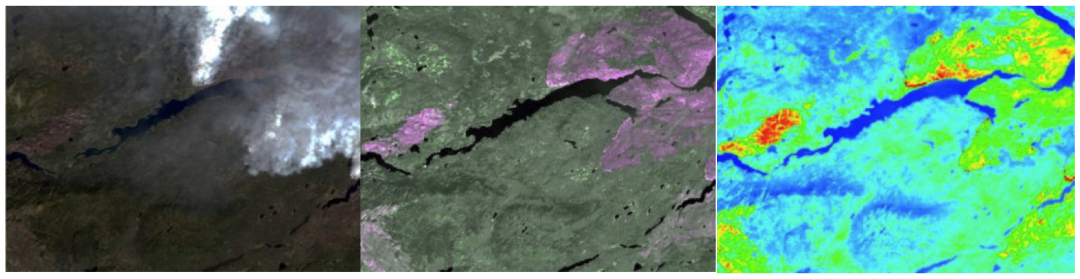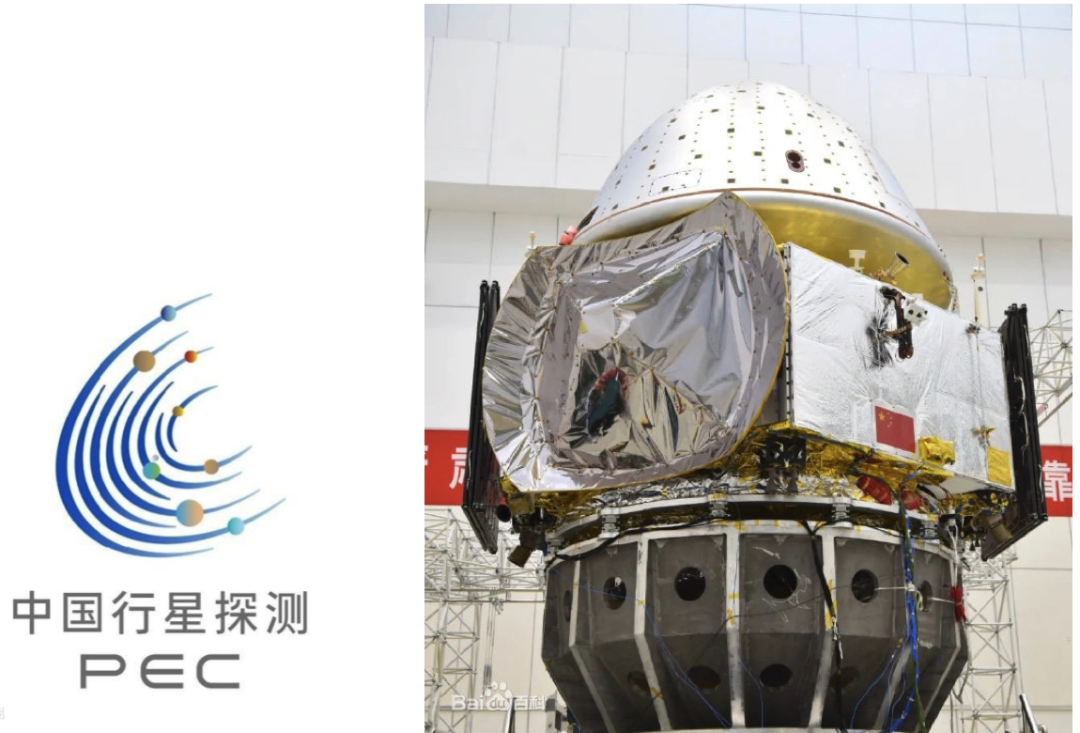```下载安装命令

## CPU版本安装命令

## GPU版本安装命令

## 本次使用的数据集是前段时间跟一位博士师哥学习时使用的数据，是师哥辛辛苦苦从各地采样带回实验室化验以及处理遥感图像得来的。我们常用的遥感卫星有高分一号、高分二号、Landsat7 、Landsat8等。以Lansat8为例，其OLI陆地成像仪包括9个波段，空间分辨率为30米，其中包括一个15米的全色波段，成像宽幅为185x185km。热红外传感器TIRS包括2个单独的热红外波段，分辨率100米。以下是各波段的常见用途。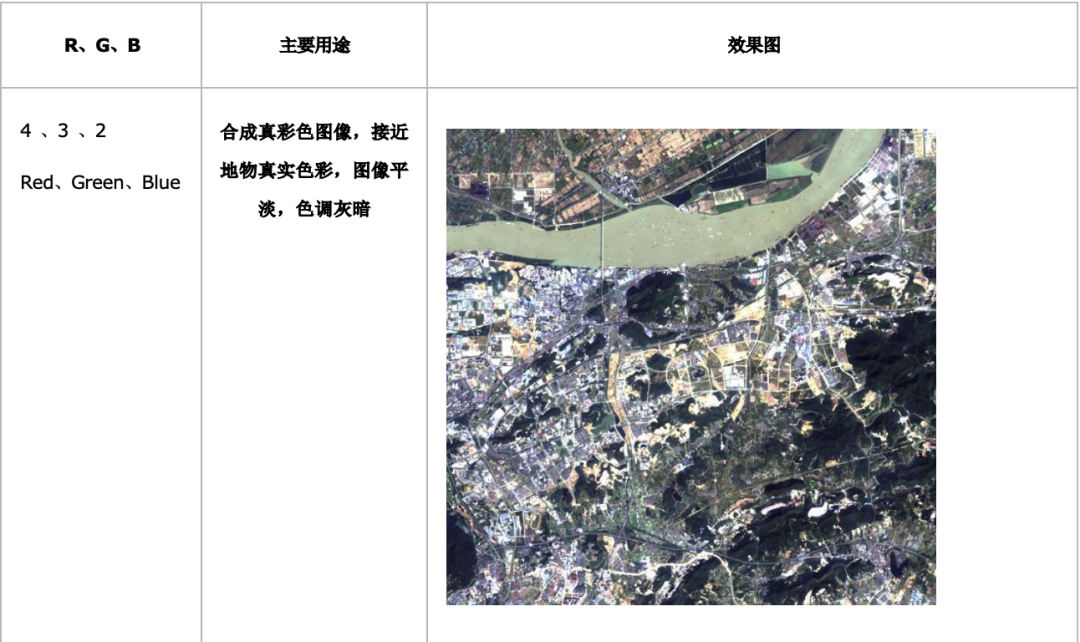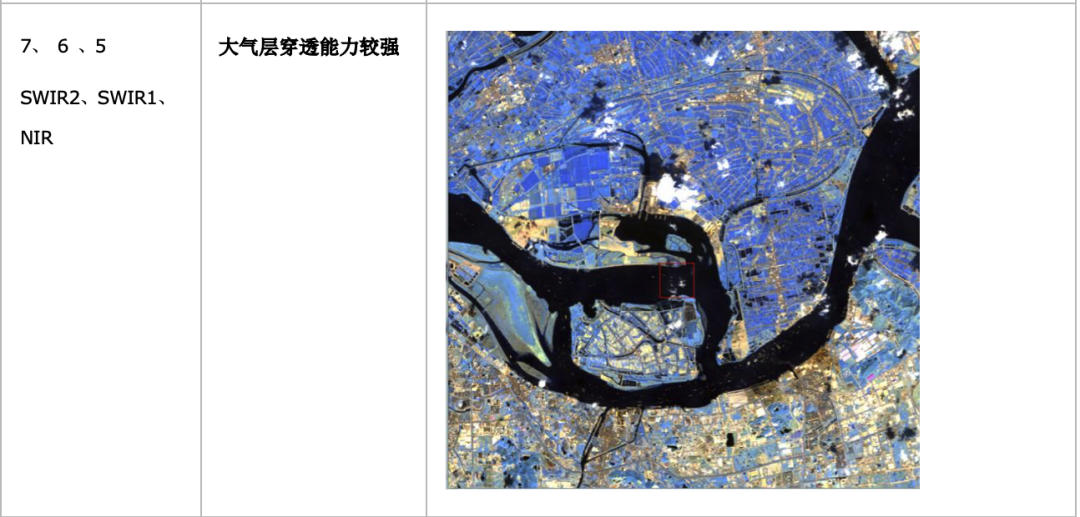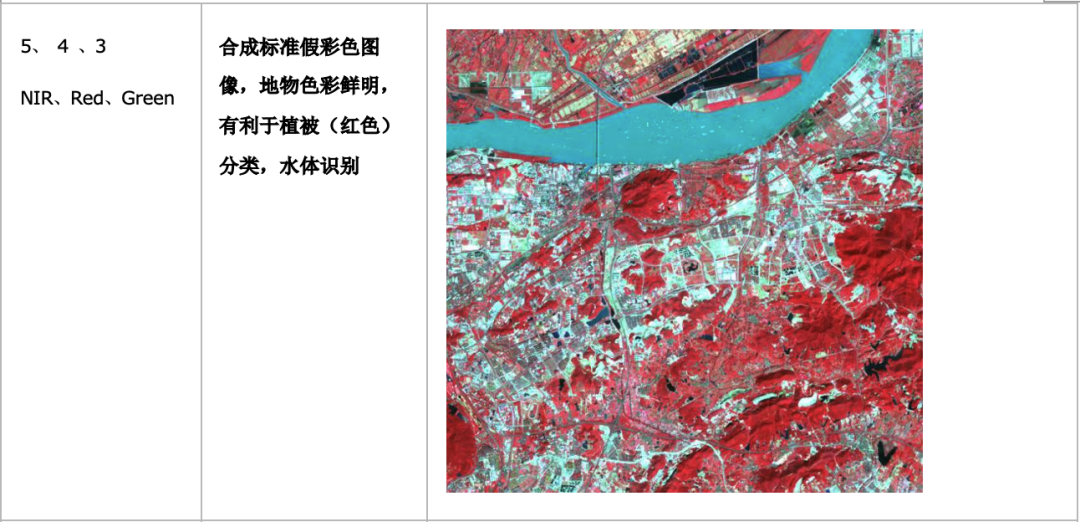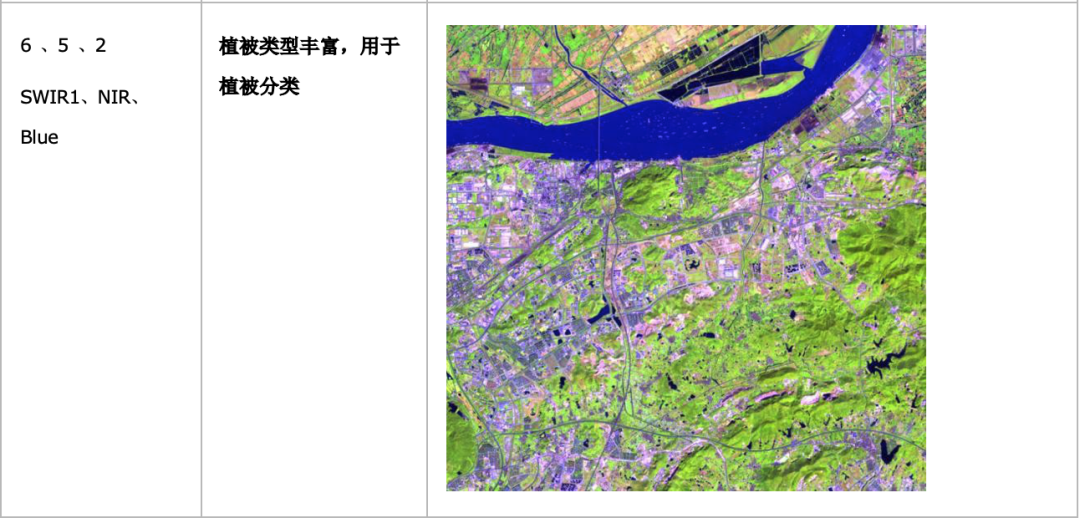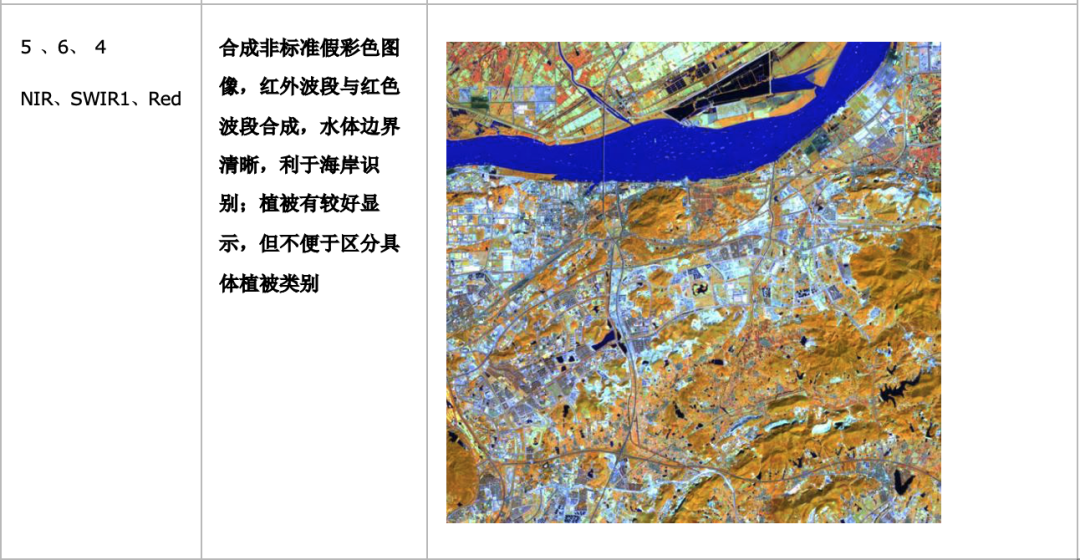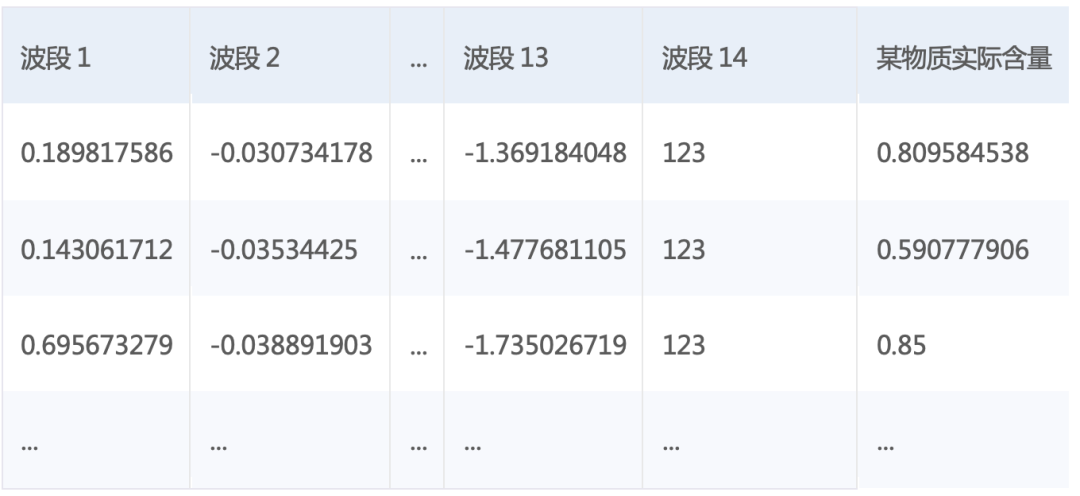https://aistudio.baidu.com/aistudio/projectdetail/693750/

``````dataset = pd.read_csv('data/data48548/data.csv')#读取数据集
# df转array
values = dataset.values
# 原始数据标准化，为了加速收敛
scaler = MinMaxScaler(feature_range=(0, 1))
scaled = scaler.fit_transform(values)
data = scaled

ratio = 0.8 # 训练集和验证集的划分比例
offset = int(data.shape*ratio)
train_data = data[:offset]
test_data = data[offset:]

for d in train_data:
yield d[:-1], d[-1:]

BUF_SIZE=50
BATCH_SIZE=20

#用于训练的数据提供器，每次从缓存中随机读取批次大小的数据
buf_size=BUF_SIZE),
batch_size=BATCH_SIZE)
#用于测试的数据提供器，每次从缓存中随机读取批次大小的数据
buf_size=BUF_SIZE),
batch_size=BATCH_SIZE)
``````

## 下面我们开始配置训练程序，目的是定义一个训练模型的网络结构。

``````#定义张量变量x，表示14维的特征值
x = fluid.layers.data(name='x', shape=, dtype='float32')
#定义张量y,表示1维的目标值
y = fluid.layers.data(name='y', shape=, dtype='float32')
#定义一个简单的线性网络,连接输入和输出的全连接层
#input:输入tensor;
#size:该层输出单元的数目
#act:激活函数
y_predict=fluid.layers.fc(input=x,size=1,act=None)

cost = fluid.layers.square_error_cost(input=y_predict, label=y) #求一个batch的损失值
avg_cost = fluid.layers.mean(cost)

optimizer = fluid.optimizer.SGDOptimizer(learning_rate=0.001)
opts = optimizer.minimize(avg_cost)
``````

## 请使用如下代码开始训练，其中EPOCH_NUM为模型的训练轮数，这个数量要适中，否则可能发生过拟合等问题

model_save_dir：模型的保存目录，保存下来，下次就可以继续训练或者直接推理了。

``````for pass_id in range(EPOCH_NUM):                                  #训练EPOCH_NUM轮
# 开始训练并输出最后一个batch的损失值
train_cost = 0
train_cost = exe.run(program=fluid.default_main_program(),#运行主程序
feed=feeder.feed(data),              #喂入一个batch的训练数据，根据feed_list和data提供的信息，将输入数据转成一种特殊的数据结构
fetch_list=[avg_cost])
if batch_id % 40 == 0:
print("Pass:%d, Cost:%0.5f" % (pass_id, train_cost))    #打印最后一个batch的损失值
iter=iter+BATCH_SIZE
iters.append(iter)
train_costs.append(train_cost)

# 开始测试并输出最后一个batch的损失值
test_cost = 0
test_cost= exe.run(program=test_program, #运行测试cheng
feed=feeder.feed(data),               #喂入一个batch的测试数据
fetch_list=[avg_cost])                #fetch均方误差
print('Test:%d, Cost:%0.5f' % (pass_id, test_cost))     #打印最后一个batch的损失值
``````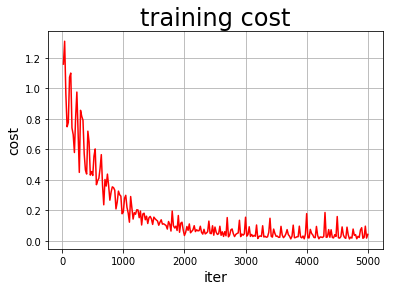## 需要构建一个使用训练好的模型来进行预测的程序，训练好的模型位置在 model_save_dir 。

``````with fluid.scope_guard(inference_scope):#修改全局/默认作用域（scope）, 运行时中的所有变量都将分配给新的scope。
#从指定目录中加载 推理model(inference model)
[inference_program,                             #推理的program
feed_target_names,                             #需要在推理program中提供数据的变量名称
model_save_dir, #model_save_dir:模型训练路径
infer_exe)      #infer_exe: 预测用executor
#获取预测数据
batch_size=20)                #从测试数据中读取一个大小为20的batch数据
test_x = np.array([data for data in test_data]).astype("float32")
test_y= np.array([data for data in test_data]).astype("float32")
results = infer_exe.run(inference_program,                #预测模型
feed={feed_target_names: np.array(test_x)},  #输入光谱数据
fetch_list=fetch_targets)            #得到推测含量

``````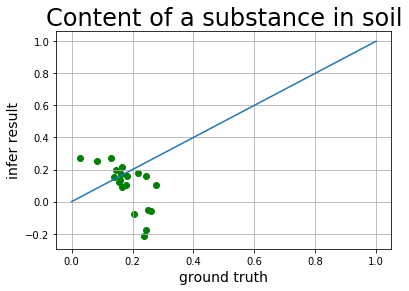https://aistudio.baidu.com/aistudio/projectdetail/693750/

```下载安装命令

## CPU版本安装命令

## GPU版本安装命令
```

GitHub:

Gitee:

0
0 收藏

### 作者的其它热门文章0 评论
0 收藏
0